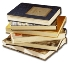Make the Best Gambling DecisionsOdds of Winning at Table GamesWhat are the OddsHourly Loss Rates by Casino Game
Odds Vs Probability -- What is the Difference?
Did You Know Odds are Better Online?Maintaining the glitzy building on the Las Vegas Strip costs a large fortune. Who pays for this? You do because the house edge on the Strip is high! Since playing online entails no brick and mortar glitz, the house edge is lower and your odds of winning increase.
Play Now at Bodog Online!

# Odds vs Probability -- What is the Difference?

The terms odds and probability are used in our everyday lives, but most people confuse them as they think they have the same meaning. Although they are related concepts, odds and probability are veryThere are occasions when we here at ReadyBetGo want to bring you interesting facts about the gambling industry  When something catches our eye, we will publish it for your enjoyment.  different in scale and meaning.

One of the areas where these two terms are used most often is in the gambling industry. As you may know, online gambling has become extremely popular in the past couple of years and online casinos became very popular. People access them on both their desktop and mobile devices

A subsidiary of the gambling industry is the world of sports betting. Some research has shown that people that like to gamble are also fans of sports betting. That is exactly the area that we wanted to focus on when detailing everything that you should know about odds and probability. So, without any further ado, let’s begin breaking down the details.

Odds – Meaning

Odds are used to determine the likelihood of a certain event to happen. For example, if you have a bag with 5 blue and 7 red balls, the odds of drawing a blue ball are 5:7. The odds are most commonly expressed as odds for, so the odds of getting yourself a blue ball here would be 0.71. How did we get that number? Simple. All you have to do is divide the number of blue balls with the number of red balls. 5 divided by 7 equals 0.71.

On the flip side, instead of expressing odds for, you can also express odds against. In that case, the process of dividing would be the opposite. Instead of dividing the number of blue balls with the number of red balls, you flip them. In this case, the odds against pulling a blue ball would be 7 divided by 5 which equals 1.4.

Understanding odds for and odds against might seem like an easy task, but they are a bit more complicated than you would think. If you would like to educate yourself on this topic, we can help you. We found an article which explains odds for vs odds against in the best possible way. Make sure you give it a read.

It is also worth noting that sports odds are represented differently in several parts of the world. There are three main types: decimal, fractional, and American. Decimal odds are the ones that we used to explain how odds work above and they are most commonly used in Europe.

Fractional odds are most commonly used in the UK and the numbers are separated by a slash. The number on the right is the stake, while the number on the left is your potential win. For example, 5/1 bet means that for every \$1 that you bet, you have the chance to win \$5.

American odds are a bit difficult for the rest of the world. They display positive and negative numbers to indicate the likelihood of an outcome. This format uses \$100 as a baseline guide. If the odds are -300, that means that you need to stake \$300 to win \$100. If the odds are +300, that means that if you bet \$100, you will win \$300.

Probability - Meaning

Probability is defined as the number of occurrences of a certain event expressed as a proportion of all events that could take place. Let’s say that you have a bag with a total of 10 balls, 5 of which are white. The probability of pulling a white ball out of the bag is 5 divided by 10, which is 50%, or 0.5.

Probabilities will always range between 0 and 1. 0 represents impossibility, while 1 represents certainty. As you can see, there are two main ways to express probabilities – with decimals and percentages.

In many circumstances, probability can be ca be determined precisely, but that is not the case with sports betting. Since many factors are involved in sports games, it is impossible to determine the precise probability of a certain outcome.

Finally, we wanted to mention two terms connected to probability – implied probability and expected value. Implied probability is what the odds suggest the likelihood of an outcome happening is. It is calculated by dividing 1 to the decimal odds.

Expected value relates to how much you can expect to win from a wager. This is a theoretical measure that is based on the overall probability of it winning. The calculation goes: Expected Value = (probability of winning multiplied with the amount won per bet) – (probability of losing multiplied with the stake).

As you can see, understanding probability is a bit complicating, which is why bookies do not use them. Its difficulty combined with the factor that is impossible to determine sports outcomes is the reason why bookies always use odds.